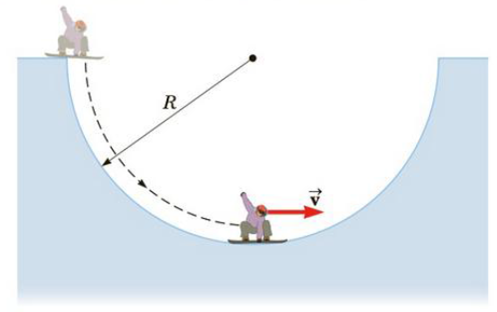Chapter 7, Problem 28P

Chapter
Section
Textbook Problem

A snowboarder drops from rest into a halfpipe of radius R and slides down its frictionless surface to the bottom (Fig. P7.28). Show that (a) the snowboarder’s speed at the bottom of the halfpipe is v = 2 gr (Hint: Use conservation of energy), (b) the snowboarder’s centripetal acceleration at the bottom is ac = 2g, and (c) the normal force on the snow-boarder at the bottom of the halfpipe has magnitude 3mg (Hint: Use Newton’s second law of motion).Figure P7.28

(a)

To determine
The speed of the snowboarder at the bottom of the half line pipe is 2gR .

Explanation

Given Info:

The radius of the frictional less half line pipe is R.

Explanation:

Consider h=0 at the bottom of the pipe.

Since, the pipe is frictionless; according to conservation of energy,

(KE+PE)i=(KE+PE)f

Since, the snowboarder is at rest initially; the kinetic energy of the snowboarder is zero. The bottom of the pipe is considered as the h=0 point. Thus, the final potential energy of the snowboarder is zero

(b)

To determine
The centripetal acceleration of the snowboarder at the bottom of the pipe is,

ac=2g .

(c)

To determine
The magnitude of the normal force on the snowboarder at the bottom of the half pipe is 3mg .

Still sussing out bartleby?

Check out a sample textbook solution.

See a sample solution

The Solution to Your Study Problems

Bartleby provides explanations to thousands of textbook problems written by our experts, many with advanced degrees!

Get Started

Find more solutions based on key concepts